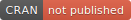# mobForest### mobForest R Package

`mobForest` implements random forest method for model based recursive partitioning. The `mob()` function, developed by Zeileis et al (2008), within party package, is modified to construct model-based decision trees based on random forests methodology. The main input function `mobforest.analysis()` takes all input parameters to construct trees, compute out-of-bag errors, predictions, and overall accuracy of forest. The algorithm performs parallel computation using `clusterApply()` function within the parallel package.

### Installation

``install.packages("mobForest")``

### Usage

To run the example, you will need the `mlbench` package. It contains a boston housing dataset for machine learning algorithms to run benchmark tests on.

``````library(mlbench)
set.seed(1111)
# Random Forest analysis of model based recursive partitioning load data
data("BostonHousing", package = "mlbench")
BostonHousing <- BostonHousing[1:90, c("rad", "tax", "crim", "medv", "lstat")]

# Recursive partitioning based on linear regression model medv ~ lstat with 3 trees.  1 core/processor used.
rfout <- mobforest.analysis(as.formula(medv ~ lstat), c("rad", "tax", "crim"),
mobforest_controls = mobforest.control(ntree = 3, mtry = 2, replace = TRUE,
alpha = 0.05, bonferroni = TRUE, minsplit = 25), data = BostonHousing,
processors = 1, model = linearModel, seed = 1111)
rfout``````